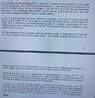# Shape of an oldie acid

• hexanimal

#### hexanimal

Homework Statement
Finding dimension of molecule and avagadros constant
Relevant Equations
Na = N/n
v = lwh
I have done all of the questions apart from c and d. For a I got the answer 2.58*10^_5 cm^3
B) 3.72*10^-7 cm
But for c I don’t really know how to start. I first thought of using the formula for volume of cuboid but don’t know how that’d give the answer for the dimension.
For D, I turned the density from 890 kg m^-3 to 0.89 g cm^-3, found the molecular mass to be 282 and from my previous question used the volume v = 2.58*10^-5 to get mass = 2.2962*10^-5g from the formula p =m/v.
I’d just like to know how to do c and d, thanks for your help

#### Attachments

•71CCD00E-2B18-4329-B9E4-5278E5A79F74.jpeg
92 KB · Views: 37
hexanimal said:
Homework Statement:: Finding dimension of molecule and avagadros constant
Relevant Equations:: Na = N/n
v = lwh

But for c I don’t really know how to start.
Consider one molecule. How is it oriented on the water? Where are other molecules in relation to it?